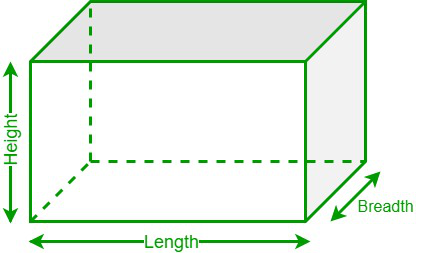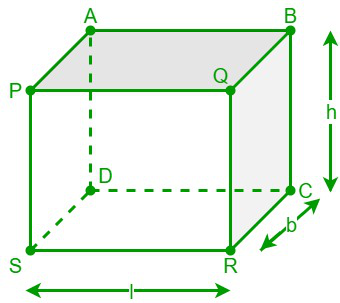# Surface Area of a Cuboid Formula

• Last Updated : 30 Jun, 2022

In geometry, a three-dimensional shape having six rectangular faces is called a cuboid. A cuboid is also known as a regular hexahedron and has six rectangular faces, eight vertices, and twelve edges with congruent opposite faces. It is a three-dimensional form of a rectangle with four lateral faces and two faces at the top and bottom. Some examples of cuboids that we regularly see are bricks, geometric boxes, shoe boxes, packaging boxes, etc. A cuboid becomes a cube if all the faces of the cuboid are square.### Surface area of a cuboid

The surface area of any three-dimensional object is called the total space occupied by all its surfaces. The lateral and total surface areas of a cuboid are the two types of surface areas of a cuboid. The lateral surface area of a cuboid is the area occupied by its lateral faces, whereas the total surface area of a cuboid is the area occupied by all its six rectangular faces.

Let us consider, that “l” is the length of the cuboid, “b” is the breadth of the cuboid, and “h” is the height of the cuboid.

Lateral surface area of a cuboid = 2h(l + b) square units

Total surface area of a cuboid = 2 (lb + bh + lh) square units

### Derivation of the surface area of a cuboid

Let us consider, that “l” is the length of the cuboid, “b” is the breadth of the cuboid, and “h” is the height of the cuboid.We know that in a cuboid, opposite faces are equal, i.e., ABCD = PQRS, APSD = BQRC, and ABQP = DCRS.

Now, the total surface area of the cuboid is equal to the sum of the areas of its six rectangular faces.

The total surface area of the cuboid = Area of [ABCD + APSD + ABQP + PQRS + BQRS + DCRS].

Area of [ABCD +APSD + ABQP + ABCD +APSD + ABQP] = 2 Area of [ABCD +APSD + ABQP]

The area of the rectangle ABCD = l × h

The area of the rectangle APSD = b × h

The area of the rectangle ABQP = l × b

Therefore,

Total surface area of the cuboid = 2 (lb + bh + lh) square units

The lateral surface area of a cuboid is the area occupied by its lateral faces.

Hence, the lateral surface area of the cuboid = The total surface area of the cuboid – The area of the top and bottom faces of the cuboid

= 2 (lb + bh + lh) – Area of [ABPQ + CDRS}

= 2 (lb + bh + lh) – 2 Area of (ABPQ)

= 2 (lb + bh + lh) – 2lb

= 2 (bh + lh) = 2h(l + b)

Hence,

Lateral surface area of a cuboid = 2h(l + b) square units

### Determining the surface area of the cuboid

Let us consider an example to determine the surface area of the cuboid using its formula.

Example: Calculate the total surface area of a cuboid if its length, breadth, and height are 8 cm, 3 cm, and 5 cm, respectively.

Solution:

Step 1: Note down the dimensions of the given sphere. Here, the length, breadth, and height are 8 cm, 3 cm, and 5 cm.

Step 2: We know that the total surface area of a cuboid = 2 (lb + bh + lh). So, substitute the value of the given dimensions in the equation = 2 [(8 × 3) + (3 × 5) + (8 × 5)] = 158 sq. cm.

Step 3: Hence, the total surface area of a cuboid is 158 sq. cm.

### Sample Problems

Problem 1: Determine the lateral surface area of a cuboid if its length, breadth, and height are 15 in, 8 in, and 12 in, respectively.

Solution:

Given data,

The length of a cuboid (l) = 15 in

The breadth of a cuboid (b) = 8 in

The height of a cuboid (h) = 12 in

We have,

Lateral surface area of a cuboid = 2h(l + b)

= 2 × 12 (15 + 8)

= 24 × 23 = 552 square inches.

Hence, the lateral surface area of the given cuboid is 552 square inches.

Problem 2: What is the total surface area of a cuboid if its length, breadth, and height are 18 cm, 13 cm, and 15 cm, respectively?

Solution:

Given data,

The length of a cuboid (l) = 18 cm

The breadth of a cuboid (b) = 13 cm

The height of a cuboid (h) = 15 cm

We have,

Total surface area of a cuboid = 2 (lb + bh + lh)

= 2 [(18 × 13) + (13 × 15) + (18 × 15)]

= 2 [234 + 195 + 270]

= 2  = 1398 cm2

Hence, the total surface area of the given cuboid is 1398 cm2.

Problem 3: Calculate the height of the cuboid whose lateral surface area is 360 square units and whose length and breadth are 12 units and 8 units, respectively.

Solution:

Given data,

The length of a cuboid (l) = 12 units

The breadth of a cuboid (b) = 8 units

The lateral surface area = 360 square units

We have,

Lateral surface area of a cuboid = 2h(l + b)

⇒ 2h (12 + 8) = 360

⇒ h × (20) = 360/2 = 180

⇒ 20h = 180⇒ h = 180/20

⇒ h = 9 units

Hence, the height of the given cuboid is 9 units.

Problem 4: Determine the length and the total surface area of a cuboid whose lateral surface area is 960 sq. in and whose breadth and height are 12 in and 16 in, respectively.

Solution:

Given data,

The breadth of a cuboid (b) = 12 in

The height of a cuboid (h) = 16 in

The lateral surface area = 960 square inches

We know that,

Lateral surface area of a cuboid = 2h(l + b)

⇒ 2 × 16 (l + 12) = 960

⇒ 32 (l + 12) = 960

⇒ (l + 12) = 960/32 = 30

⇒ l = 30 – 12 = 18 in

We have,

Total surface area of a cuboid = 2 (lb + bh + lh)

= 2 [(18 × 12) + (12 × 16) + (18 × 16)]

= 2 [ 216 + 192 + 288]

= 2 ×  = 1398 square inches

Hence, the length and the total surface area of the cuboid are 18 in and 1398 sq. in, respectively.

Problem 5: Calculate the total surface area of a cuboid if its length, breadth, and height are 10 in, 5 in, and 8 in, respectively.

Solution:

Given data,

The length of a cuboid (l) = 10 in

The breadth of a cuboid (b) = 5 in

The height of a cuboid (h) = 8 in

We have,

Total surface area of a cuboid = 2 (lb + bh + lh)

= 2 [(10 × 5) + (5 × 8) + (10 × 8)]

= 2 × [50 + 40 + 80]

= 2 × (170) = 340 sq. in

Hence, the total surface area of the given cuboid is 340 sq. in.

Problem 6: Determine the lateral and total surface areas of a cuboid whose length, breadth, and height are 21 cm, 16 cm, and 18 cm, respectively.

Solution:

Given data,

The length of a cuboid (l) = 21 cm

The breadth of a cuboid (b) = 16 cm

The height of a cuboid (h) = 18 cm

We have,

Lateral surface area of a cuboid = 2h(l + b)

= 2 × 18 (21 + 16)

= 36 × 37 = 1332 sq. cm

Total surface area of a cuboid = 2 (lb + bh + lh)

= 2 [(21 × 16) + (16 × 18) + (21 × 18)]

= 2 [336 + 288+ 378] = 2 × 1002

= 2004 sq. cm

Therefore, the lateral surface area and the total surface area of a cuboid are 1332 sq. cm and 2004 sq. cm, respectively.

My Personal Notes arrow_drop_up
Recommended Articles
Page :